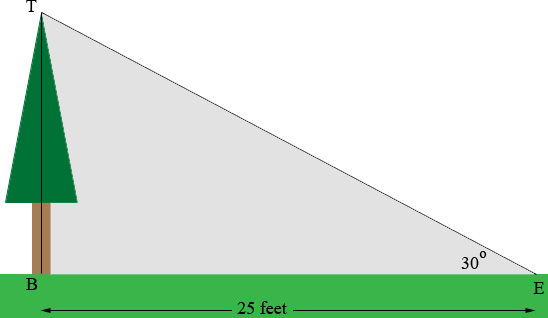SEARCH HOMEMath Central Quandaries & QueriesQuestion from danice, a student: At a certain time of day, the angle of elevation of the sun is 30°. A tree has a shadow that is 25 feet long. Find the height of the tree to the nearest foot.Danice,T is the top of the tree, B is the bottom of the tree and E is the end of the shadow. Triangle TBE is a right triangle so what trig function relates the measure of the angle BET (30o), the length of BE (25 feet) and the length of TB? Solve for the length of TB, the height of the tree.

PennyMath Central is supported by the University of Regina and The Pacific Institute for the Mathematical Sciences.